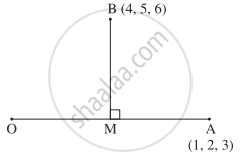# If A(1, 2, 3) and B(4, 5, 6) are two points, then find the foot of the perpendicular from the point B to the line joining the origin and the point A. - Mathematics and Statistics

Sum

If A(1, 2, 3) and B(4, 5, 6) are two points, then find the foot of the perpendicular from the point B to the line joining the origin and the point A.

#### SolutionLet M be the foot of the perpendicular drawn from B to the line joining O and A.

Let M = (x, y, z)

OM has direction ratios x - 0, y - 0, z - 0 = x, y, z

OA has direction ratios 1 - 0, 2 - 0, 3 - 0 = 1, 2, 3

But O, M, A are collinear.

∴ "x"/1 = "y"/2 = "z"/3 = "k"  ....(Let)

∴ x = k, y = 2k, z = 3k

∴  m = (k, 2k, 3k)

BM has direction ratios

k - 4, 2k - 5, 3k - 6

∵ BM is perpendicular to OA.

∴ (1)(k - 4) + 2(2k - 5) + 3(3k - 6) = 0

∴ k - 4 + 4k - 10 + 9k - 18 = 0

∴ 14k = 32

∴ k = 16/7

∴ M = (k, 2k, 3k) = (16/7, 32/7, 48/7)

#### Notes

[Note: Answer in the textbook is incorrect.]

Concept: Vector Product of Vectors (Cross)
Is there an error in this question or solution?